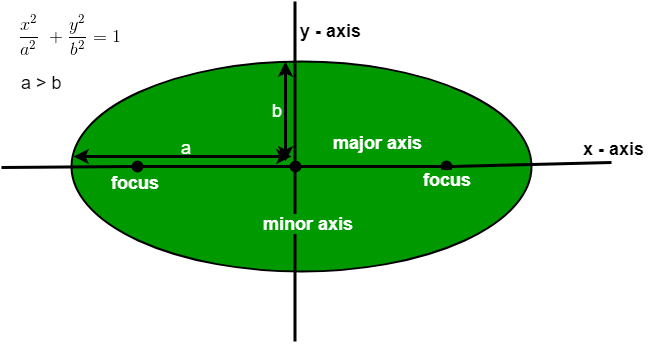Program to find the Area of an Ellipse

• Last Updated : 06 Apr, 2021

Given an ellipse with a semi-major axis of length a and semi-minor axis of length b. The task is to find the area of an ellipse.
In mathematics, an ellipse is a curve in a plane surrounding by two focal points such that the sum of the distances to the two focal points is constant for every point on the curve or we can say that it is a generalization of the circle.Important points related to Ellipse

• Center: A point inside the ellipse which is the midpoint of the line segment which links the two foci. In other words, it is the intersection of minor and major axes.
• Major Axis: The longest diameter of an ellipse is termed as the major axis.
• Minor Axis: The shortest diameter of an ellipse is termed as minor axis.
• Chord: A line segment that links any two points on an ellipse.
• Focus: These are the two fixed points that define an ellipse.
• Latus Rectum: The line segments which passes through the focus of an ellipse and perpendicular to the major axis of an ellipse, is called as the latus rectum of an ellipse.

Area of an ellipse: The formula to find the area of an ellipse is given below:

Area = 3.142 * a * b

where a and b are the semi-major axis and semi-minor axis respectively and 3.142 is the value of π.
Examples:

Input : a = 5, b = 4
Output : 62.48

Input : a = 10, b = 5
Output : 157.1

C++

 // C++ program to find area of// an Ellipse.#includeusing namespace std; // Function to find area of an// ellipse.void findArea( float a, float b){    float Area;         // formula to find the area    // of an Ellipse.    Area = 3.142 * a * b ;         // Display the result    cout << "Area: " << Area;} // Driver codeint main(){    float a = 5, b = 4;         findArea(a, b);         return 0;}

Java

 // Java program to find area of// an Ellipse.class GFG {     // Function to find area of an    // ellipse.    static void findArea( float a, float b)    {        float Area;                 // formula to find the area        // of an Ellipse.        Area = (float)3.142 * a * b ;                 // Display the result        System.out.println("Area: " + Area);    }         // Driver code    public static void main (String[] args)    {        float a = 5, b = 4;                 findArea(a, b);    }}

Python3

 # Python3 program to find# area of an Ellipse. # Function to find area# of an ellipse.def findArea(a, b):         # formula to find the    # area of an Ellipse.    Area = 3.142 * a * b ;         # Display the result    print("Area:", round(Area, 2)); # Driver codea = 5;b = 4; findArea(a, b); # This code is contributed# by mits

C#

 // C# program to find area of// an Ellipse.using System;class GFG{     // Function to find area    // of an ellipse.    static void findArea(float a,                         float b)    {        float Area;                 // formula to find the        // area of an Ellipse.        Area = (float)3.142 * a * b ;                 // Display the result        Console.WriteLine("Area: " +                           Area);    }         // Driver code    public static void Main ()    {        float a = 5, b = 4;                 findArea(a, b);    }} // This code is contributed// by anuj_67.



Javascript


Output:
Area: 62.84

My Personal Notes arrow_drop_up